Need Help?

Subscribe to Circuit

###### \${selected_topic_name}
• Notes
• Comments & Questions

$\text { Find the equivalent capacitance between a, b. }$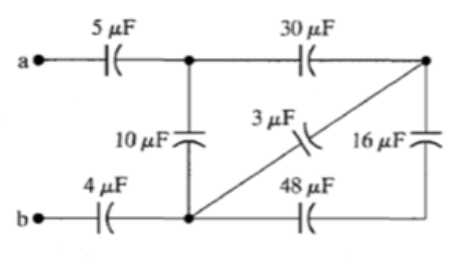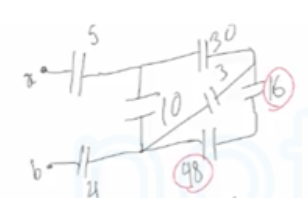$C_{eq}=\frac{c_{1} c_{2}}{c_{1}+c_{2}}=\frac{98*16}{98+16}=12 \mu F$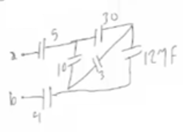$C_{eq}=C_{1}+C_{2}=12+3=15 \mu F$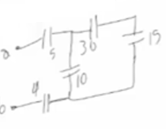$C_{eq}=\frac{C_{1} C_{2}}{C_{1}+C_{2}}=\frac{15 * 30}{15+30}=10 \mathrm{\mu F}$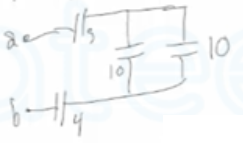$C_{eq}=C_{1}+C_{2}=10+10=20 \mu F$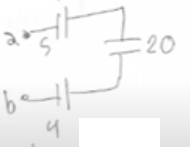$\frac{1}{c_{eq}}=\frac{1}{c_{1}}+\frac{1}{c_{2}}+\frac{1}{c_{3}}$

$\frac{1}{c_{eq}}=\frac{1}{20}+\frac{1}{4}+\frac{1}{5}$

$c_{eq}=2 \mu F$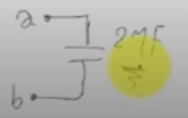No comments yet